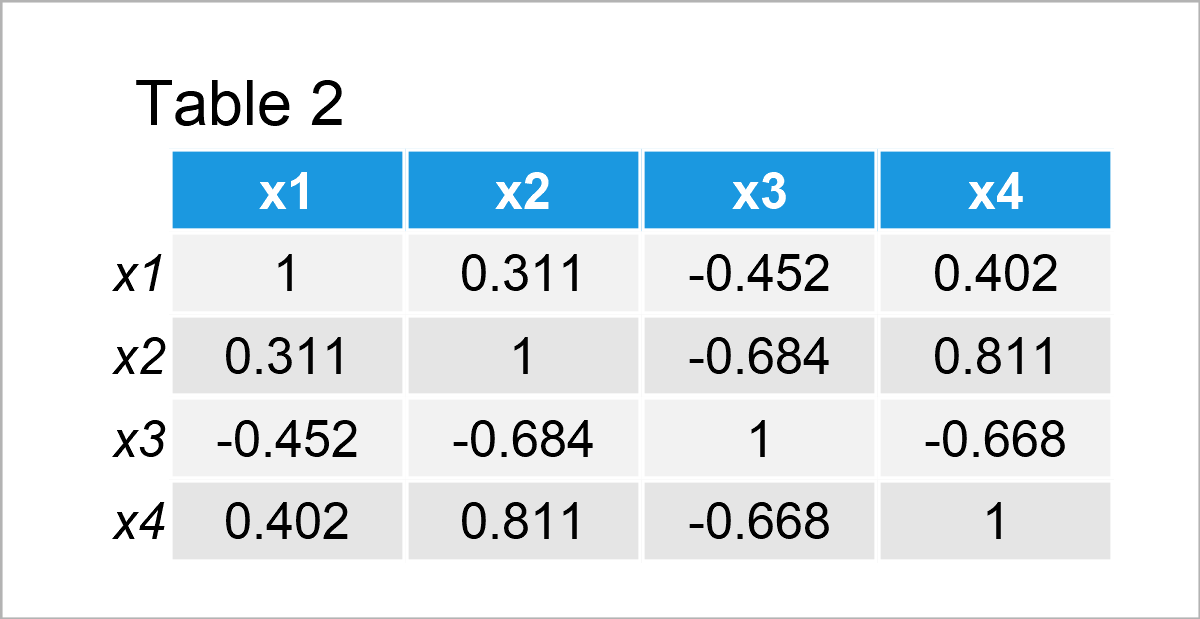# Remove Diagonal from Correlation Matrix Plot in R (2 Examples)

This article explains how to eliminate the diagonal from a correlation matrix plot in the R programming language.

The tutorial will consist of two examples for the elimination of the diagonal from a correlation matrix plot. To be more specific, the tutorial is structured as follows:

Let’s jump right to the exemplifying R syntax:

## Example Data

Let’s first construct some example data:

```set.seed(41651)                # Create example data
x1 <- rnorm(200)
x2 <- rnorm(200) + 0.3 * x1
x3 <- runif(200) - 0.1 * x1 - 0.25 * x2
x4 <- runif(200) + 0.1 * x1 + 0.3 * x2 - 0.25 * x3
data <- data.frame(x1, x2, x3, x4)The previous table shows that our example data consists of four numerical variables.

Next, we can create a correlation matrix of these data using the cor function:

```data_cor <- cor(data)          # Create correlation matrix
data_cor                       # Print correlation matrix```After running the previous syntax the correlation matrix shown in Table 2 has been created.

Let’s draw these data!

## Example 1: Eliminate Diagonal from Correlation Matrix Plot Created by corrplot Package

This example demonstrates how to create a correlation matrix plot without the diagonal using the corrplot package.

First, we have to install and load the corrplot package:

```install.packages("corrplot")   # Install & load corrplot
library("corrplot")```

Next, we can apply the corrplot function to draw our correlations. Note that we are setting the diag argument to be equal to FALSE to avoid drawing the diagonal of our correlation matrix:

```corrplot(data_cor,             # corrplot graphic without diagonal
diag = FALSE)```Figure 1 shows the output of the previously shown R syntax – A corrplot correlation matrix plot without a diagonal.

## Example 2: Eliminate Diagonal from Correlation Matrix Plot Created by ggcorrplot Package

In Example 2, I’ll demonstrate how to create a correlation matrix plot with ggplot2 style using the ggcorrplot package.

To be able to use the functions of the ggcorrplot package, we first need to install and load ggcorrplot:

```install.packages("ggcorrplot") # Install ggcorrplot package

Next, we have to set the diagonal of our correlation matrix to NA.

```data_cor_NA <- data_cor        # Create duplicate of correlation matrix
diag(data_cor_NA) <- NA        # Replace diagonal by NA
data_cor_NA                    # Print updated correlation matrix```After running the previous code the new correlation matrix without diagonal values shown in Table 3 has been created.

Now, we can apply the ggcorrplot function to draw a correlation matrix plot without diagonal:

`ggcorrplot(data_cor_NA)        # ggcorrplot graphic without diagonal`## Video, Further Resources & Summary

In case you need more information on the R syntax of this post, you may watch the following video on my YouTube channel. In the video, I illustrate the topics of this article:

Please accept YouTube cookies to play this video. By accepting you will be accessing content from YouTube, a service provided by an external third party.If you accept this notice, your choice will be saved and the page will refresh.

Furthermore, you might want to have a look at the other R tutorials on my website.

Summary: You have learned in this tutorial how to delete the diagonal from a correlation matrix graphic in the R programming language. Kindly let me know in the comments, in case you have any additional questions.

Subscribe to the Statistics Globe Newsletter